# manim.mobject.svg.tex_mobject¶

Mobjects representing text rendered using LaTeX.

Note

Just as you can use Text (from the module text_mobject) to add text to your videos, you can use Tex and MathTex to insert LaTeX.

## The Tex mobject¶

Example: HelloLaTeX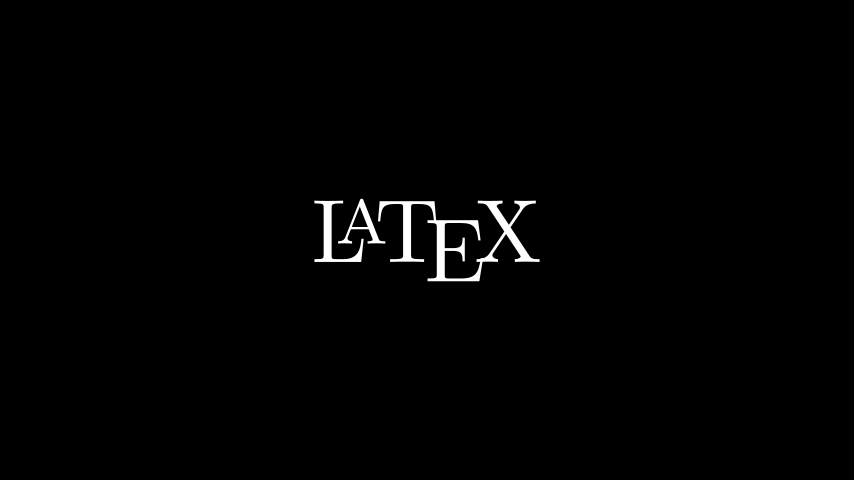from manim import *

class HelloLaTeX(Scene):
def construct(self):
tex = Tex(r'\LaTeX').scale(3)
self.add(tex)


Note that we are using a raw string (r'---') instead of a regular string ('---'). This is because TeX code uses a lot of special characters - like \ for example - that have special meaning within a regular python string. An alternative would have been to write \\ as in Tex('\\LaTeX').

Anything enclosed in $ signs is interpreted as maths-mode: Example: HelloTexfrom manim import * class HelloTex(Scene): def construct(self): tex = Tex(r'$\xrightarrow{x^2y^3}$\LaTeX').scale(3) self.add(tex)  Whereas in a MathTex mobject everything is math-mode by default. Example: MovingBraces from manim import * class MovingBraces(Scene): def construct(self): text=MathTex( "\\frac{d}{dx}f(x)g(x)=", #0 "f(x)\\frac{d}{dx}g(x)", #1 "+", #2 "g(x)\\frac{d}{dx}f(x)" #3 ) self.play(Write(text)) brace1 = Brace(text, UP, buff=SMALL_BUFF) brace2 = Brace(text, UP, buff=SMALL_BUFF) t1 = brace1.get_text("$g'f$") t2 = brace2.get_text("$f'g$") self.play( GrowFromCenter(brace1), FadeIn(t1), ) self.wait() self.play( ReplacementTransform(brace1,brace2), ReplacementTransform(t1,t2) ) self.wait()  ## LaTeX commands and keyword arguments¶ We can use any standard LaTeX commands in the AMS maths packages. For example the mathtt math-text type, or the looparrowright arrow. Example: AMSLaTeX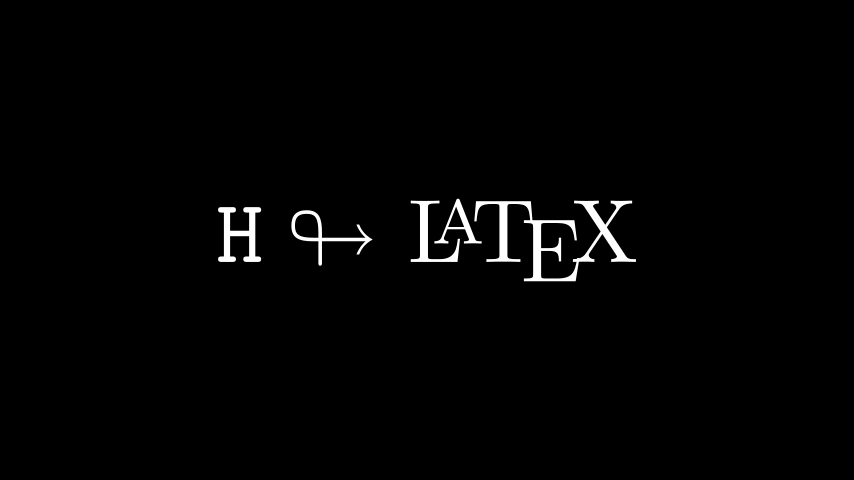from manim import * class AMSLaTeX(Scene): def construct(self): tex = Tex(r'$\mathtt{H} \looparrowright$\LaTeX').scale(3) self.add(tex)  On the manim side, the Tex class also accepts attributes to change the appearance of the output. This is very similar to the Text class. For example, the color keyword changes the color of the TeX mobject: Example: LaTeXAttributes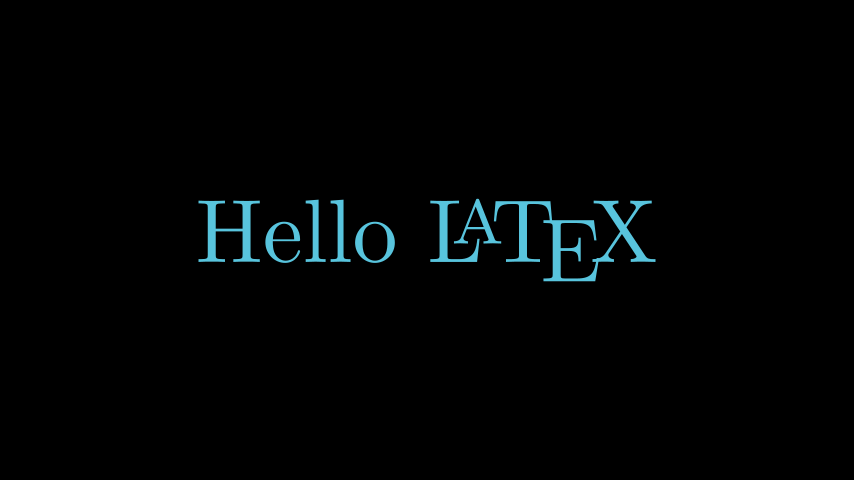from manim import * class LaTeXAttributes(Scene): def construct(self): tex = Tex(r'Hello \LaTeX', color=BLUE).scale(3) self.add(tex)  ## Extra LaTeX Packages¶ Some commands require special packages to be loaded into the TeX template. For example, to use the mathscr script, we need to add the mathrsfs package. Since this package isn’t loaded into manim’s tex template by default, we add it manually: Example: AddPackageLatex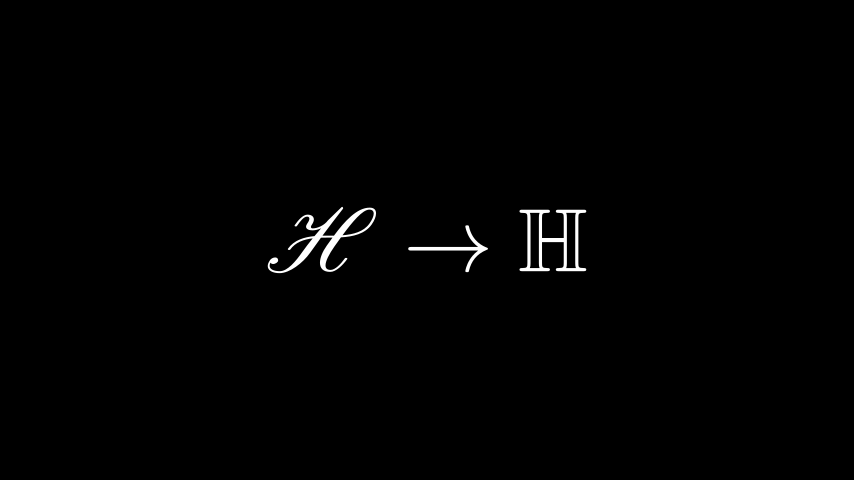from manim import * class AddPackageLatex(Scene): def construct(self): myTemplate = TexTemplate() myTemplate.add_to_preamble(r"\usepackage{mathrsfs}") tex = Tex(r'$\mathscr{H} \rightarrow \mathbb{H}$}', tex_template=myTemplate).scale(3) self.add(tex)  ## Substrings and parts¶ The TeX mobject can accept multiple strings as arguments. Afterwards you can refer to the individual parts either by their index (like tex), or you can look them up by (parts of) the tex code like in this example where we set the color of the \bigstar using set_color_by_tex(): Example: LaTeXSubstringsfrom manim import * class LaTeXSubstrings(Scene): def construct(self): tex = Tex('Hello', r'$\bigstar$', r'\LaTeX').scale(3) tex.set_color_by_tex('igsta', RED) self.add(tex)  Note that set_color_by_tex() colors the entire substring containing the Tex searched for, not just the specific symbol or Tex expression searched for. Consider the following example: Example: IncorrectLaTeXSubstringColoringfrom manim import * class IncorrectLaTeXSubstringColoring(Scene): def construct(self): equation = MathTex( r"e^x = x^0 + x^1 + \frac{1}{2} x^2 + \frac{1}{6} x^3 + \cdots + \frac{1}{n!} x^n + \cdots" ) equation.set_color_by_tex("x", YELLOW) self.add(equation)  As you can see, this colors the entire equation yellow, contrary to what may be expected. To color only x yellow, we have to do the following: Example: CorrectLaTeXSubstringColoring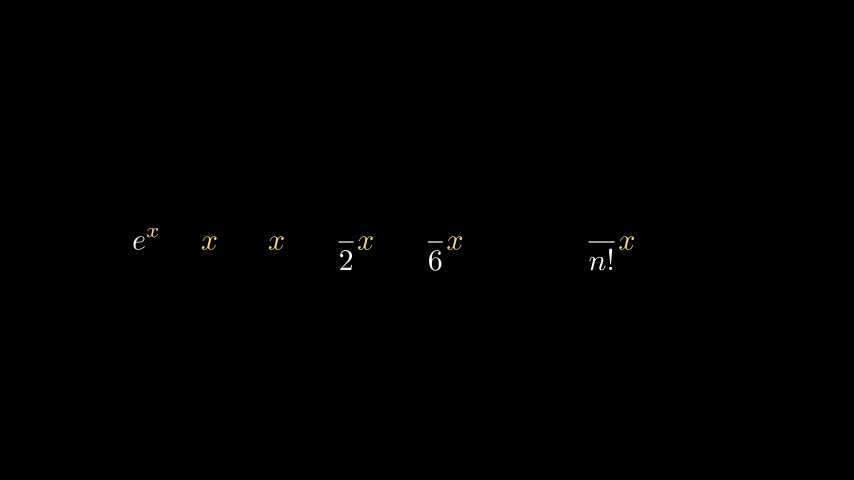from manim import * class CorrectLaTeXSubstringColoring(Scene): def construct(self): equation = MathTex( r"e^x = x^0 + x^1 + \frac{1}{2} x^2 + \frac{1}{6} x^3 + \cdots + \frac{1}{n!} x^n + \cdots", substrings_to_isolate="x" ) equation.set_color_by_tex("x", YELLOW) self.add(equation)  By setting substring_to_isolate to x, we split up the MathTex into substrings automatically and isolate x components into individual substrings. Only then can set_color_by_tex() be used to achieve the desired result. ## LaTeX Maths Fonts - The Template Library¶ Changing fonts in LaTeX when typesetting mathematical formulae is a little bit more tricky than with regular text. It requires changing the template that is used to compile the tex code. Manim comes with a collection of TexFontTemplates ready for you to use. These templates will all work in maths mode: Example: LaTeXMathFonts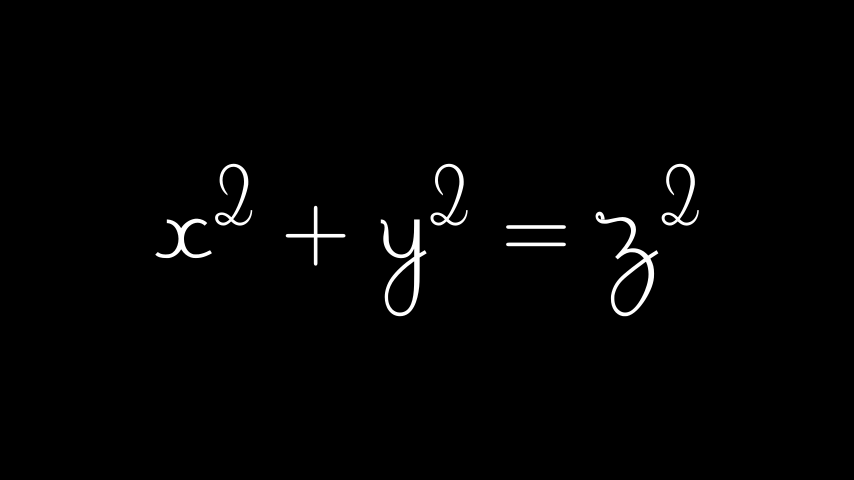from manim import * class LaTeXMathFonts(Scene): def construct(self): tex = Tex(r'$x^2 + y^2 = z^2\$', tex_template=TexFontTemplates.french_cursive).scale(3)
self.add(tex)


Manim also has a TexTemplateLibrary containing the TeX templates used by 3Blue1Brown. One example is the ctex template, used for typesetting Chinese. For this to work, the ctex LaTeX package must be installed on your system. Furthermore, if you are only typesetting Text, you probably do not need Tex at all, and should use Text or PangoText instead.

Example: LaTeXTemplateLibrary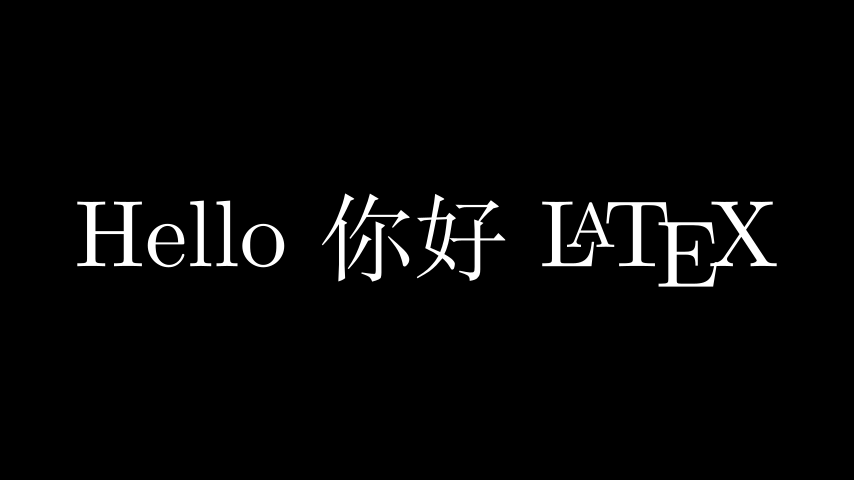from manim import *

class LaTeXTemplateLibrary(Scene):
def construct(self):
tex = Tex('Hello 你好 \\LaTeX', tex_template=TexTemplateLibrary.ctex).scale(3)
self.add(tex)


## Aligning formulae¶

A MathTex mobject is typeset in the LaTeX align* environment. This means you can use the & alignment character when typesetting multiline formulae:

Example: LaTeXAlignEnvironment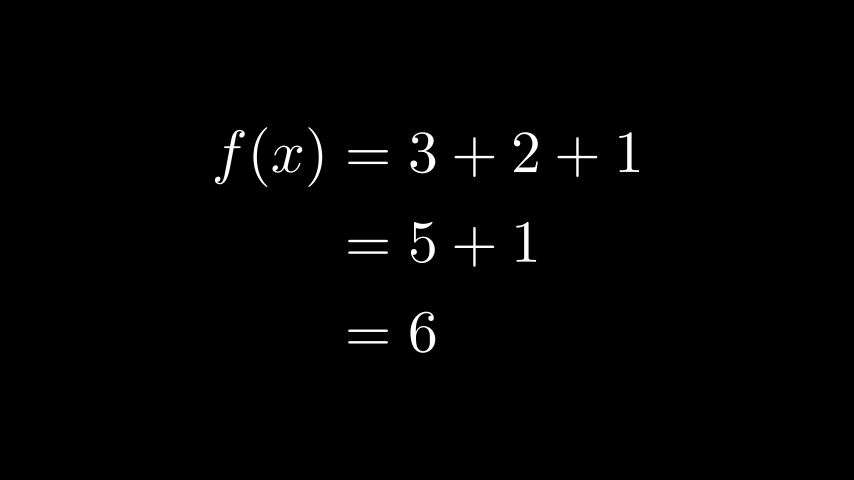from manim import *

class LaTeXAlignEnvironment(Scene):
def construct(self):
tex = MathTex(r'f(x) &= 3 + 2 + 1\\ &= 5 + 1 \\ &= 6').scale(2)
self.add(tex)


Classes

 BulletedList MathTex A string compiled with LaTeX in math mode. SingleStringMathTex Elementary building block for rendering text with LaTeX. Tex A string compiled with LaTeX in normal mode. TexSymbol Purely a renaming of SVGPathMobject. Title Examples